The Moon and its Orbit

Objective:

In this activity the student will investigate the Orbit of the Moon.

Background:

As the Earth orbits the Sun, as seen from the Earth the Sun traces out a path in the sky that is called the Ecliptic. When studying the orbit of the Moon and eclipses it is convenient to define a new coordinate system that is referenced to the plane of the Ecliptic as shown in (figure1).  The position of the Sun and Moon in the sky as viewed from Earth is depicted.

Ecliptic longitude is measured along the Ecliptic in degrees starting from the position of the Sun on the first day of spring in March.  This point is called the First Point of Aires. The Ecliptic longitude of the Sun increases approximately one degree per day as the Sun moves eastward along the Ecliptic. Ecliptic latitude locates an object north or south of the Ecliptic.

Eclipses

The Moon orbits the Earth in a plane that is inclined to the plane of the Ecliptic at an angle of approximately 5 degrees, as depicted in (figure 1).  When the Moon has the same Ecliptic longitude as the Sun it is called a New Moon. When the Moon has an Ecliptic longitude greater than the Sun’s by 180 degrees we call it a Full Moon. It takes approximately 29 ½ days for the Moon to go through all its phases and become a New Moon again. Twice every lunar orbit the Moon crosses the Ecliptic. These two points are known as the Ascending Node and Descending Node.

Both the Earth and the Moon cast shadows into space. When the shadow of the Moon falls onto the Earth a Solar Eclipse occurs. When the shadow of the Earth falls onto the Moon a Lunar Eclipse occurs. For a Solar Eclipse to occur, the Moon must cross the Ecliptic within 18.5 degrees of the Sun’s current Ecliptic longitude at New Moon. This region that guarantees an Eclipse is called the Eclipse Limits as shown in (Figure 1).  Every six months the Moon crosses the Ecliptic within the Eclipse Limits and an eclipse occurs.  Figure1 depicts a solar eclipse that occurred on Mar 18 as the Moon crossed the ascending node. In months other than March it can the Moon will pass above or below the Sun at each New Moon. In August the Sun is again near a node and eclipses are again possible.

An interesting property of eclipses is that nearly identical eclipse occurs every18 years 11 days and 8 hours. This occurs because the line of nodes rotates in a westward direction taking 18 years to complete a full rotation.  In the example discussed later eclipses will occur in Feb and July due to the rotation of the line of nodes.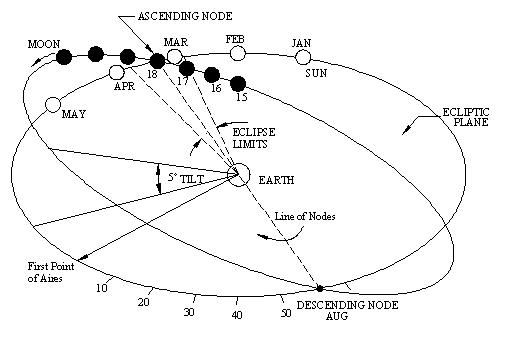Figure 1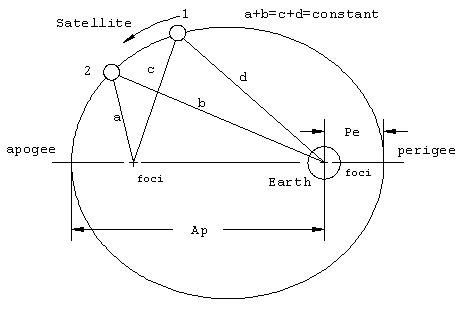Figure 2

The Moons Orbit

When a Satellite orbits the Earth the orbit will be an ellipse (Figure 2). An ellipse has the property that the sum of the distance of the orbiting body from the two foci of the ellipse is a constant.  The Earth is located at one foci as shown in figure 2. The point of closest approach is called perigee (Pe), and the point of furthest distance is called apogee (Ap).

Eccentricity measures how elongated an orbit is. The higher the eccentricity the more elongated the ellipse. A circle has an eccentricity of zero.

The eccentricity can be calculated from the Apogee and Perigee distance of the orbiting body with the following formula.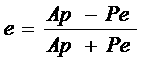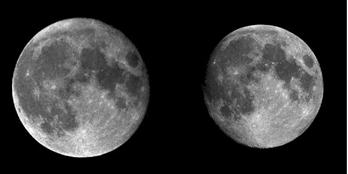Moon at Perigee                      Moon at Apogee

Figure 3

As the Moon orbits the Earth its distance from the Earth changes, and so does its observed angular size.  The pictures in Figure 3 were taken with the same telescope with the same magnification on different dates. The change in angular diameter of the Moon is quite obvious. The change in Angular size is directly proportional to the distance of the Moon. If the Moon was twice as close to the Earth it would appear twice as big in the sky. We will use this change in angular diameter to calculate the relative eccentricity of its orbit.

Over the period of a simulated month, we will measure the angular size and position of the Moon using the Sky software.  We will measure the Ecliptic longitude and Ecliptic latitude of the Moon for each observation.  The Ecliptic latitude will tell us whether the Moon is above or bellow the plane of the Ecliptic. From this we can find the Ecliptic longitude of both the Ascending and Descending node. We will then find the dates for the eclipse seasons and calculate the eccentricity of the moon’s orbit.

Procedure:

Using the Sky Software and locate the moon on the given dates.  Set the date and time as indicated and face the indicated direction.  The moon should appear on your screen.  Click on the moon to open up the information window.   In the window, click on the expand button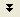to view the full information window.  Then change all degree-minute readings to decimals by clicking the conversion button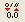.   Figure 4 shows the data for November 20, 2007 at 3PM.

The ecliptic coordinates are given in the line labeled Ecliptic.  The first number (following the l:) is the ecliptic longitude.  The second number, (following the b:) is the ecliptic latitude.  Record ecliptic longitude to the nearest degree and the ecliptic latitude to one decimal place.  For the example displayed we would record the Ecliptic Longitude as 7 degrees and the ecliptic latitude as 2.8 degrees.

Record the angular diameter of the moon to three decimal places.  So in the example, record the angular diameter as 0.545 degrees.

Finally, when recording the “moon distance”, round to the nearest 1000 km.  In other words 365,413km is rounded to 365,000 km.  When recording the data in the table, just record the number of thousands of kilometers.  In this case, 365.Figure 4

ECLIPSE SEASONS

On the graph provided, plot the Ecliptic Longitude and Ecliptic Latitude of the moon for each day.  Draw a smooth curve connecting the points.  Find the nodes of the moons orbit (the points where the moon’s path crosses the ecliptic) and record the Ecliptic Longitude of each node on your data sheet.

Now estimate on what days on which the Sun will be located at one of the nodes.  To do this estimate each month having 30 days and the Sun increasing its ecliptic longitude by one degree each day.  So take the ecliptic longitude of the node and divide by 30 noting the remainder.  The number is the number of months past March 21 and the remainder is the number of days following.

For example, if a node is located at an ecliptic longitude of 135°.  Then we first divide by 30 and get an answer of 4 with a remainder of 15.  Which means that the Sun will located at this node 4 months and 15 days after March 21.  The three months get us to June 21.  Then add 15 days to get July 6.

MOON’S ORBIT

Next we’ll find the eccentricity of the moon’s orbit.  Examine the columns of angular diameter and moon distance.  How are the two related?

Under the column of distances record the largest number as the apogee (Ap) and the shortest distance as perigee (Pe) then use the given equation to calculate the eccentricity of the moon’s orbit.

Choose three distances from the list, Apogee, Perigee, and one in between.  For these dates multiply the angular size by the distance.  What do you observe?